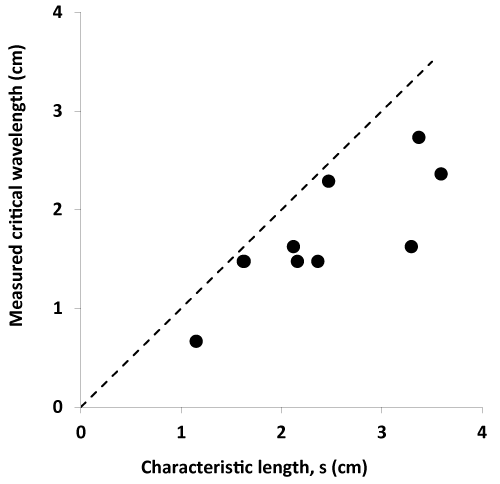Figure 13: Comparison of measured critical impulse wavelengths required for VT/VT and the characteristic linear dimensions of abnormal, ischemic tissue zones. Characteristic linear dimensions, s, are calculated as the cube roots of ischemic tissue volumes. The dashed line at 45 degrees is the line of equality (y=x). These data are uncorrected for flattening.